ConicBundle
ConicBundle::SparseCoeffmatMatrix Class Reference

stores/organizes the CoeffmatPointer pointers to Coeffmat matrices with the purpose of describing the block diagonal symmetric matrices used in PSCAffineFunction More...

#include <SparseCoeffmatMatrix.hxx>

Inheritance diagram for ConicBundle::SparseCoeffmatMatrix:## Public Types

typedef std::map< CH_Matrix_Classes::Integer, SparseCoeffmatVectorSCMcolrep
this will be used for a (lazy) column representation of the matrix

## Public Member Functions

SparseCoeffmatMatrixoperator= (const SparseCoeffmatMatrix &)
copy

void clear ()
clears all (empty 0 times 0 matrix)

int init (const CH_Matrix_Classes::Indexmatrix &block_dim, CH_Matrix_Classes::Integer col_dim, const CH_Matrix_Classes::Indexmatrix *block_ind=0, const CH_Matrix_Classes::Indexmatrix *col_ind=0, const CoeffmatVector *coeff_vec=0)
first calls clear() and then it sets the new values (if one of block_ind, col_ind, or coeff_vec is !=NULL, all must be !=NULL and of the same size)

SparseCoeffmatMatrix (const CBout *cb=0, int incr=-1)
set the output and call clear()

SparseCoeffmatMatrix (const SparseCoeffmatMatrix &S, const CBout *cb=0, int incr=-1)
set the output and call clear()

SparseCoeffmatMatrix (const CH_Matrix_Classes::Indexmatrix &in_block_dim, CH_Matrix_Classes::Integer in_col_dim, const CH_Matrix_Classes::Indexmatrix *block_ind=0, const CH_Matrix_Classes::Indexmatrix *col_ind=0, const CoeffmatVector *coeff_vec=0, const CBout *cb=0, int incr=-1)
set the output and call init() for the given sparse information

~SparseCoeffmatMatrix ()
calls clear() and exits

const CH_Matrix_Classes::Indexmatrixblockdim () const
returns a column vector with row i giving the order of block i

CH_Matrix_Classes::Integer blockdim (CH_Matrix_Classes::Integer i) const
returns the order of block i

CH_Matrix_Classes::Integer coldim () const
returns the number of columns (i.e. of blockdiagonal matrices)

CH_Matrix_Classes::Integer rowdim () const
returns the number of blocks

CH_Matrix_Classes::Integer nzcoldim () const
returns the number of columns with nonzero coefficient matrices

int set (CH_Matrix_Classes::Integer i, CH_Matrix_Classes::Integer j, const CoeffmatPointer &cm)
sets the CoeffmatPointer of block i in column j (blockdiagonal matrix j) (may be empty)

int set (CH_Matrix_Classes::Integer i, CH_Matrix_Classes::Integer j, Coeffmat *cm)
sets the CoeffmatPointer of block i in column j (blockdiagonal matrix j) to point to cm (or deletes it if cm==0)

CoeffmatPointer operator() (CH_Matrix_Classes::Integer i, CH_Matrix_Classes::Integer j) const
returns the CoeffmatPointer of block i in column j (blockdiagonal matrix j) (may be empty)

const SparseCoeffmatVectorblock (CH_Matrix_Classes::Integer i) const
returns NULL if the block is empty and a pointer to a Vector of CoeffmatPointers otherwise

const SparseCoeffmatVectorcolumn (CH_Matrix_Classes::Integer i) const
returns NULL if the column is empty and a pointer to a Vector of CoeffmatPointers otherwise

int append_blocks (const SparseCoeffmatMatrix &append_mat, const CH_Matrix_Classes::Indexmatrix *blocks=0, const CH_Matrix_Classes::Indexmatrix *cols=0)
append append_mat (or its submatrix given by blocks and/or cols) below More...

int append_columns (const SparseCoeffmatMatrix &append_mat, const CH_Matrix_Classes::Indexmatrix *blocks=0, const CH_Matrix_Classes::Indexmatrix *cols=0)
append append_mat (or its submatrix given by blocks and/or cols) to the right More...

int reassign_blocks (const CH_Matrix_Classes::Indexmatrix &map_to_old)
afterwards the new block i is the previous block map_to_old(i); no multiple appearances are allowed, but not all have to appear (these are deleted)

int reassign_columns (const CH_Matrix_Classes::Indexmatrix &map_to_old)
afterwards the new column i is the previous column map_to_old(i); no multiple appearances are allowed, but not all have to appear (these are deleted)

int delete_blocks (const CH_Matrix_Classes::Indexmatrix &delete_indices, CH_Matrix_Classes::Indexmatrix *map_to_old=0)
generates a map_to_old by deleting the specified indices and calls reassign_blocks; this map_to_old will be returned if the corresponding pointer is not NULL

int delete_columns (const CH_Matrix_Classes::Indexmatrix &delete_indices, CH_Matrix_Classes::Indexmatrix *map_to_old=0)
generates a map_to_old by deleting the specified indices and calls reassign_columns; this map_to_old will be returned if the corresponding pointer is not NULL

const SCMcolrepget_colrep () const
returns the column representation of the matrix

CH_Matrix_Classes::Integer get_dense_cnt (CH_Matrix_Classes::Integer i) const
returns the number of dense matrices in block i

bool operator== (const SparseCoeffmatMatrix &mat) const
useful for testing purposes; true iff both have the same size and both have the same pointers in the same positions

bool operator!= (const SparseCoeffmatMatrix &mat) const
useful for testing purposes; false iff both have the same size and both have the same pointers in the same positions

int Gram_ip (CH_Matrix_Classes::Matrix &ipvec, const CH_Matrix_Classes::Matrix &P, const CH_Matrix_Classes::Matrix *Lam=0, const CH_Matrix_Classes::Indexmatrix *ind=0) const
computes the inner products of (selected) columns (which represent block diagonal symmetric matrices) with the Gram matrix P*P^T (or, if Lam is given, P*Diag(Lam)*P^T) into the column vector ipvec(j)=ip(P*P^T,A.column((*ind)(j)}) (j=0,...,ind->dim()-1); if ind==NULL, use all columns

int Gram_ip (CH_Matrix_Classes::Real &ipval, const CH_Matrix_Classes::Matrix &P, CH_Matrix_Classes::Integer j) const
computes the inner product of the block diagonal symmetric matrix stored in colummn j with the Gram matrix P*P^T into ipval=ip(P*P^T,A.column(j))

int primal_ip (CH_Matrix_Classes::Matrix &ipvec, const PSCPrimal *primal, const CH_Matrix_Classes::Indexmatrix *ind=0) const
computes the inner products of (selected) columns (which represent block diagonal symmetric matrices) with the primal into the column vector ipvec(j)=ip(*primal,A.column((*ind)(j)}) (j=0,...,ind->dim()-1); if ind==NULL, use all columns

int primal_ip (CH_Matrix_Classes::Real &value, const PSCPrimal *primal, CH_Matrix_Classes::Integer j) const
computes the inner product of the block diagonal symmetric matrix stored in colummn j with the primal into ipval=ip(*primal,A.column(j))

int project (CH_Matrix_Classes::Symmatrix &S, const CH_Matrix_Classes::Matrix &P, const CH_Matrix_Classes::Integer j) const
computes S=P^T*A.column(j)*PPublic Member Functions inherited from ConicBundle::CBout
virtual void set_out (std::ostream *out=0, int print_level=1)
Specifies the output level (out==NULL: no output at all, out!=NULL and level=0: errors and warnings, level>0 increasingly detailed information) More...

virtual void set_cbout (const CBout *cb, int incr=-1)
Specifies the output level relative to the given CBout class. More...

void clear_cbout ()
reset to default settings (out=0,print_level=1)

CBout (const CBout *cb=0, int incr=-1)
calls set_cbout

CBout (std::ostream *outp, int pl=1)
initialize correspondingly

CBout (const CBout &cb, int incr=0)
copy constructor

virtual bool cb_out (int pl=-1) const
Returns true if out!=0 and (pl<print_level), pl<0 should be used for WARNINGS and ERRORS only, pl==0 for usual output.

std::ostream & get_out () const
If cb_out() returned true, this returns the output stream, but it will abort if called with out==0.

std::ostream * get_out_ptr () const
returns the pointer to the output stream

int get_print_level () const
returns the print_level

virtual int mfile_data (std::ostream &out) const
writes problem data to the given outstream

## Private Types

typedef std::vector< SparseCoeffmatVectorSCMblockrep
each row/block is likely to have at least one nonzero entry, therefore the block representation uses a vector of SparseCoeffmatVector (instead of a map)

## Private Member Functions

void form_colrep () const
rebuilds the column representation from the block representation (if needed)

## Private Attributes

CH_Matrix_Classes::Indexmatrix block_dim
column vector holding for each row the dimension of the block

CH_Matrix_Classes::Integer col_dim
number of columns in the SparseCoeffmatMatrix

CH_Matrix_Classes::Indexmatrix dense_cnt
number of dense matrices per block

SCMblockrep blockrep
this is the basic block representation, it holds for each row/block a sparse representation of the row via a SparseCoeffmatVector; this is the real work horse

SCMcolrepcolrep
this column representation is only formed on demand and deleted on changes

## Detailed Description

stores/organizes the CoeffmatPointer pointers to Coeffmat matrices with the purpose of describing the block diagonal symmetric matrices used in PSCAffineFunction

In an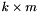SparseCoeffmatMatrix A, each row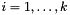describes a linear symmetric matrix function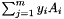of matrices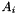of order blockdim(i). Alternatively each column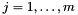of A describes a block diagonal matrix consisting of k diagonal blocks with block i of size blockdim(i).

This class offers a number of routines for modifications, like appending further blocks or columns, deleting, reassigning indices, setting single entries etc.

Likewise it allows to extract single rows or columns in the form of a SparseCoeffmatVector

For each Coeffmat coefficient matrix the matrix only stores a CoeffmatPointer pointing to it, so if the Coeffmat pointed to is changed externally, this matrix class will not notice this but simply keep pointing to the changed object.

## ◆ append_blocks()

 int ConicBundle::SparseCoeffmatMatrix::append_blocks ( const SparseCoeffmatMatrix & append_mat, const CH_Matrix_Classes::Indexmatrix * blocks = 0, const CH_Matrix_Classes::Indexmatrix * cols = 0 )

append append_mat (or its submatrix given by blocks and/or cols) below

If blocks or cols have negative entries, each of these represent a row of blocks of zeros whose order is the negative of the given value or a column of zeros of appropriate size (the size of the negative value does not matter for columns)

Referenced by set().

## ◆ append_columns()

 int ConicBundle::SparseCoeffmatMatrix::append_columns ( const SparseCoeffmatMatrix & append_mat, const CH_Matrix_Classes::Indexmatrix * blocks = 0, const CH_Matrix_Classes::Indexmatrix * cols = 0 )

append append_mat (or its submatrix given by blocks and/or cols) to the right

If blocks or cols have negative entries, each of these represent a row of blocks of zeros whose order is the negative of the given value (if columns exist already, these need to match the order of the existing blocks) or a column of zeros of appropriate size (the size of the negative value does not matter for columns)

Referenced by set().

The documentation for this class was generated from the following file: